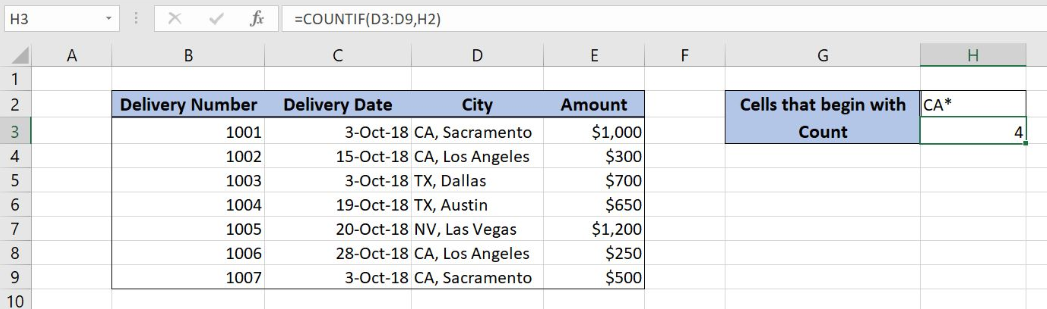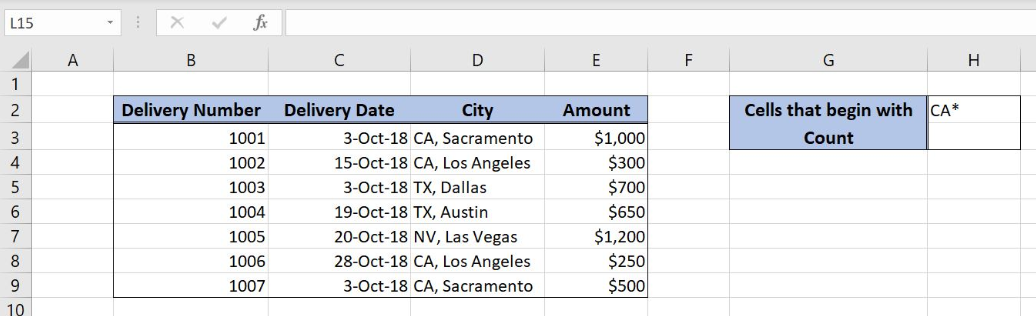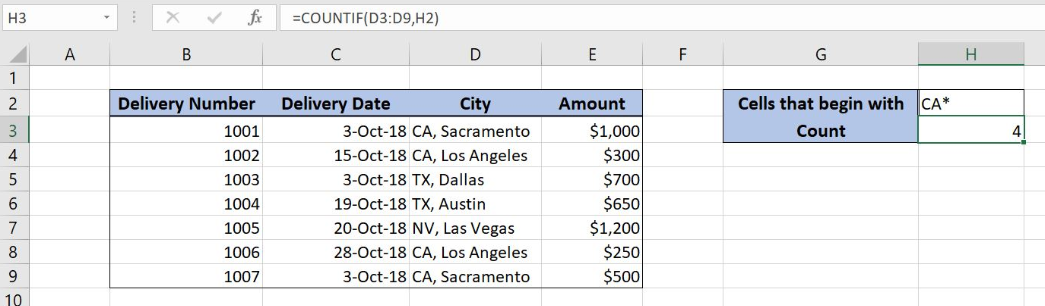Get instant live expert help with Excel or Google Sheets“My Excelchat expert helped me in less than 20 minutes, saving me what would have been 5 hours of work!”

#### Post your problem and you’ll get Expert help in seconds.

Your message must be at least 40 characters
Our professional Expert are available now. Your privacy is guaranteed.

# Count cells that begin with

Excel allows a user to count cells that begin with certain characters, by using the COUNTIF function. This step by step tutorial will assist all levels of Excel users in counting cells in a range that begin with a specific text.Figure 1. The result of the COUNTIF function

## Syntax of the COUNTIF Formula

The generic formula for the COUNTIF function is:

`=COUNTIF(criteria_range, criteria)`

The parameters of the COUNTIFS function are:

• criteria_range – a range where we want to apply our criteria
• criteria – a criteria which we want to count

## Setting up Our Data for the COUNTIF FunctionFigure 2. Data that we will use in the COUNTIF example

In the data table, we have 4 columns:  “Delivery Number” (column B), “Delivery Date” (column C), “City” (column D) and “Amount” (column E). In the cell H2, we have a criteria for the function, while in H3 we want to get a result.

## Count Cells That Begin With a Certain Text

In our example, we want to count how many cells from the column D begin with “CA”.

The formula looks like:

`=COUNTIF(D3:D9, H2)`

The parameter criteria_range is D3:D9 and the criteria is in the cell H2. We have to put an asterisk in criteria (CA*). An asterisk stands for any character.

To apply the COUNTIF function, we need to follow these steps:

• Select cell H3 and click on it
• Insert the formula: `=COUNTIF(D3:D9, H2)`
• Press enterFigure 3. Counting the cells that begins with a certain text

As you can see, cells D3, D4, D8 and D9 begin with “CA”, so the result of the function in the cell H3 is 4.

Most of the time, the problem you will need to solve will be more complex than a simple application of a formula or function. If you want to save hours of research and frustration, try our live Excelchat service! Our Excel Experts are available 24/7 to answer any Excel question you may have. We guarantee a connection within 30 seconds and a customized solution within 20 minutes.

### Did this post not answer your question? Get a solution from connecting with the expert.Another blog reader asked this question today on Excelchat:
Solution examplesI have a spreadsheet that contains a list of names/IDs and I want to generate a formula that inserts into a cell the number of times a name or ID appears on that list. Either insert a column into that spreadsheet or transfer that info into a column on a different spreadsheet. Either way would be fine.
Solved by O. L. in 18 minsI need a formula to count the number of rows that contain at least one of two possible phrases, but not give me the total number of instances that both phrases occur overall.
Solved by E. B. in 33 minsHello, I have a spreadsheet with 3159 rows. One column consists of 9-digit numbers in each of the 3159 rows. I need the formula that can calculate how many of those numbers appear more than once.
Solved by D. H. in 56 minsi need a formula to find values in column f in worksheet 1 and count them.
Solved by B. A. in 20 minsi have a table with Dates, First names , Last names and i want to count how many times a name occurs, but if a name occurs more than once on a particular date i need to only count it once. this is on excel and not google sheets
Solved by F. H. in 31 mins## Subscribe to Excelchat.coAnother blog reader asked this question today on Excelchat: